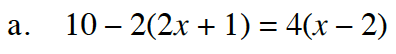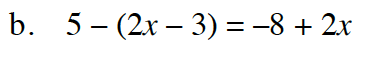Home > GB8I > Chapter cc46 > Lesson cc46.3.2 > Problem6-99

6-99.
1. Solve each equation for x. Graph your solution on a number line. Homework Help ✎

1. 10 − 2(2x + 1) = 4(x − 2)

2. 5 − (2x − 3) = −8 + 2xRemember to check your answer to make sure it is correct.

One way to start is by multiplying.

10 − 4x − 2 = 4x − 8Remember to distribute the negative.

x = 4

Simplify by adding 4x to each side.

8 = 8x − 8

Simplify again by adding 8 to both sides.

16 = 8x

Divide both sides by 8.

x = 2